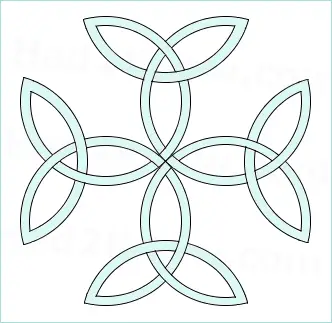# How to Draw a Carolingian Cross

A Carolingian Cross is named after the Carolingian Dynasty of France who took up the symbol for their coat of arms. Also called a Triquetra Cross, the figure is formed by arranging four Trinity Knots around a central point to form the shape of a cross. Although the knot design is Celtic pagan in origin, the symbol was later adopted by the Christian Church in Medieval Europe.

While the interlaced figure looks intricate, it is quite easy to draw in a step-by-step manner using simple geometry.

#### Tools

Graphics software or pencil, ruler, protractor, and compass.

#### Instructions

Step 1: Draw a perfect square with the exact center marked.

Step 2: Use the four vertices of the square as the centers of four circles that pass through the center of the square. (See diagram below.)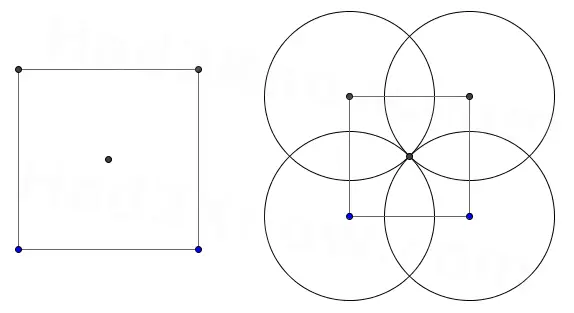Step 3: Append an equilateral triangle to each side of the square. (See diagram below.)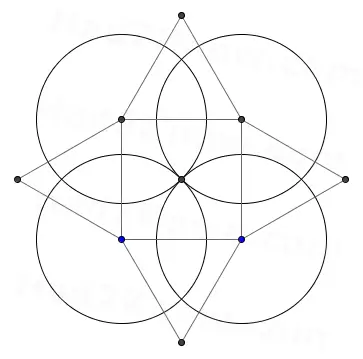Step 4: Take the outermost vertex of each of the four triangles as the centers of four new circles, each have the same radius as the original four circles. (See diagram below.)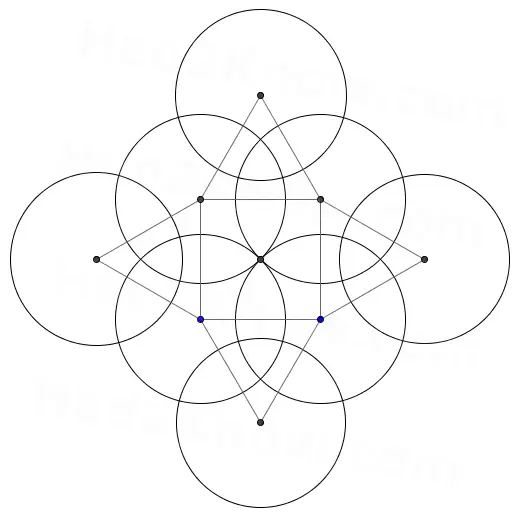Step 5: Within each of the eight circles, draw a smaller concentric circle to give the knot some thickness. (See diagram below.)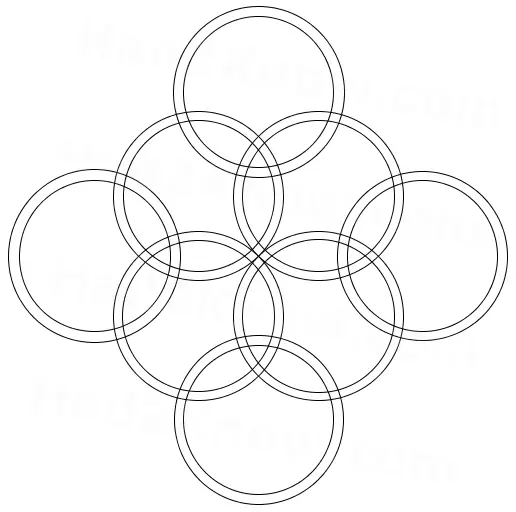Step 6: Erase the edges of the square and triangles and erase the excess arcs to reveal the Carolingian Cross. Erase the overlapping lines of the knot to give the appearance of interlacing. (See diagram below.)